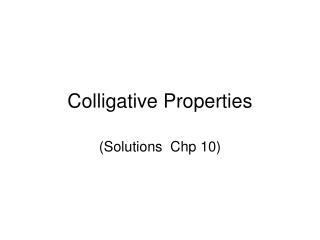# Colligative Properties - PowerPoint PPT PresentationDownload PresentationColligative Properties

Colligative PropertiesDownload Presentation## Colligative Properties

- - - - - - - - - - - - - - - - - - - - - - - - - - - E N D - - - - - - - - - - - - - - - - - - - - - - - - - - -
##### Presentation Transcript

1. Colligative Properties (Solutions Chp 10)

2. Units • Mass Percent: mass of solute/total mass of solution • Mole Fraction (X): moles/total moles • Molality (m): moles of solute/kg of solvent • Molarity (M): moles of solute/liters of solution

3. Principles of Solubility • The nature of solvent and solute particles and the interactions between them • Like dissolves like (polarity) • Most non polar have small water solubility • Ionic compounds: • Bigger force of H2O and the ions (soluble) • Bigger force of opposite charges (stays solid)

4. Principles of Solubility (cont.) • The temperature of which the solution is formed (T increased) • Endothermic rxn: more soluble • Exothermic rxn: less soluble • The pressure of a gaseous solute (g + l) • Henry’s Law : [gas] = k Pgas

5. Colligative Properties • properties of a solution that depends primarily upon the concentration of solute particles • Two types: • Nonelectrolytes (molecules in sol.) • Electrolytes (ions in sol.)

6. Nonelectrolytes • Vapor pressure lowering- vapor pressure of a solution is less than that of pure water • Raoult’s law: • P1 = X1P1o X1 = (1 – X2) • P1o –P1 = ΔP ΔP = X2P1o • Osmotic pressure & reverse osmosis • When osmotic pressure (π) is less than the external pressure (P) the solution permeates through to the pure water, reverse osmosis • π = [B]RT(

7. Nonelectrolytes (cont.) • Boiling points elevated • Δ Tb = Tb - Tbo • Freezing points lowered • ΔTf = Tfo - Tf

8. Electrolytes • When electrolytes are dissolved, the increase the concentration, intensifying the past properties • Ex. • 1 mol glucose dissolves = 1 mol • 1 mol NaCl dissolves = 2 mol • 1 mol Ca2Cl dissolves = 3 mol • Etc.